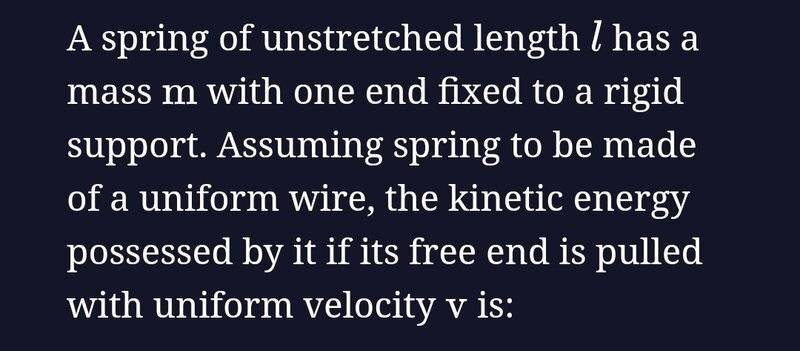# The kinetic energy of a spring

• Differentiate it
In summary, the answer key for this problem recommends using the formula for kinetic energy, but when attempting to do so, it was apparently the wrong answer. This seems to be due to the fact that an unstretched spring has a velocity, and when using the kinetic energy equation, the velocity is not accounted for.

#### Differentiate it

Homework Statement
Could someone explain the situation here?
Relevant Equations
(1/2)mv^2I tried just using the formula for kinetic energy but that was apparently the wrong answer. The answer key says it's (1/6)mv². I don't understand how they got that answer. Could someone explain?

Differentiate it said:
Yes, I tried
I think @BvU's point is that we need to see it!

••malawi_glenn and BvU
Differentiate it said:
Homework Statement:: Could someone explain the situation here?
Relevant Equations:: (1/2)mv^2

I tried just using the formula for kinetic energy
So, that would be correct for a particle, why do you think it doesn't work for a spring?

•bob012345
•Lnewqban and erobz
You have to think about what is going on physically with this spring. One end is fixed and the other is free. The free end is suddenly pulled at a velocity ##v##. You are looking at it at time zero, the free end has a velocity but hasn't actually stretched yet, it is still length ##l##. What's going on with the rest of the spring at that instant?

Last edited:
•erobz
bob012345 said:
You have to think about what is going on physically with this spring. One end is fixed and the other is free. The free end is suddenly pulled at a velocity ##v##. You are looking at it at time zero, the free end has a velocity but hasn't actually stretched yet, it is still length ##l##. What's going on with the rest of the spring at that instant?
To add to what @bob012345 is hinting at, specifically think about the fixed end. What velocity is it moving at?

•bob012345
bob012345 said:
The free end is suddenly pulled at a velocity v.
Not suddenly, I think. That would get you into complexities of internal oscillations.

haruspex said:
Not suddenly, I think. That would get you into complexities of internal oscillations.
I looked at this problem as idealized. The free end of an unstretched spring is found to be moving at velocity ##v##. Suddenly was an unfortunate choice not because of what the actual dynamics of a real spring would be since this is an ideal spring, but because there is no need to involve time at all. This is a snapshot.

bob012345 said:
since this is an ideal spring
An ideal spring is massless; this one isn't, which is why a sudden tug will likely induce internal oscillations.

haruspex said:
An ideal spring is massless; this one isn't, which is why a sudden tug will likely induce internal oscillations.
Sure, but for this problem there is only an agent pulling the free end with uniform velocity. It must be assumed that there is also a velocity distribution set up along the spring and we don't need to worry about the details of how that came about.

bob012345 said:
Sure, but for this problem there is only an agent pulling the free end with uniform velocity. It must be assumed that there is also a velocity distribution set up along the spring and we don't need to worry about the details of how that came about.
Quite so, we have to assume some sort of steady state, whatever that means in this context. Hence, no sudden movements.

•bob012345
Differentiate it said:
Homework Statement:: Could someone explain the situation here?
Relevant Equations:: (1/2)mv^2

I tried just using the formula for kinetic energy but that was apparently the wrong answer. The answer key says it's (1/6)mv². I don't understand how they got that answer. Could someone explain?
@Differentiate it, have you used the hints we gave you to make progress with this problem?